# K-Nearest Neighbors (KNN) Algorithm

K-Nearest Neighbor is a Supervised Machine Learning algorithm that can be used to solve classification as well as regression problems. It is probably the first "machine learning" algorithm developed, and because of its simple nature, it is still widely accepted in solving many industrial problems. The unique thing about this algorithm is it learns but without explicitly mapping input variables to the target variables. In this article, we are going to understand this algorithm in detail.

### Key Takeaways from this blog

• What is the KNN algorithm in Machine Learning?
• Why is KNN instance-based learning or a Lazy learner?
• Why KNN is a non-parametric algorithm?
• What are the common assumptions in KNN?
• How does KNN work?
• How the value of K affects the KNN algorithm?
• How does feature scaling affect KNN?
• What are the Voronoi cell and Voronoi diagrams?
• KNN for regression problems.
• Implementation of the KNN algorithm in python.

### What is the KNN algorithm in Machine Learning?

In the introduction section, we already have explained KNN formally. Now, let's understand it in layman's terms. Some friends did not understand the concepts in our school days and still scored well in exams because of their memorization skills. We can correlate those friends with KNN. This ML algorithm does not follow the traditional approach of learning parameters from the training data and tries to fit a function. Instead, it memorizes the complete training data instances, and whenever a new test sample comes, it tries to verify the similarity of the test sample with its learned training samples.

### Why is KNN instance-based learning or a Lazy learner?

Instance-based learning is also known as memory-based learning. Instead of explicit generalization, KNN compares new data samples with training data samples present in its memory.

They are also called lazy algorithms, as any computation only happens when we receive new observations. Before accepting any test sample, it just memorizes everything in its memory and defers the calculations for the last like a lazy person.

### Why KNN is a non-parametric algorithm?

KNN comes under the non-parametric algorithm category. Can we guess why? It is learning the complete training set, so if there are more instances in the future, the learning will change drastically. Hence learning is not dependent on the given data, which is a characteristic of a non-parametric algorithm.

### What are the common assumptions in KNN?

This algorithm makes two major assumptions:

• Every sample part of the training data is mapped to real n-dimensional space. We can say that every sample will have the same dimension or number of attributes in simple terms.
• The "nearest neighbors" are defined in terms of Euclidean Distance, Manhattan Distance, or Hamming Distance. The choice of distance matters a lot and can change the prediction.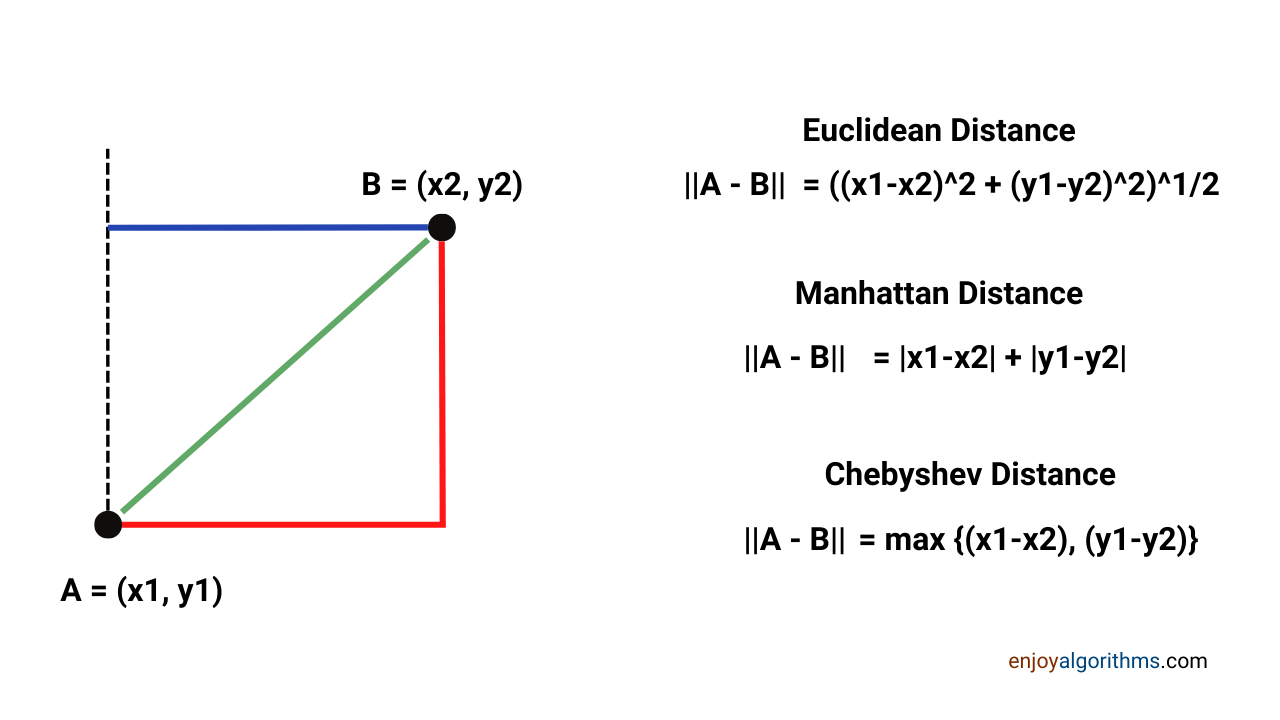### Working of KNN

Let's understand the stepwise analysis of this algorithm for any classification problem.

Step1: We first need to select the number of neighbors we want to consider. This is the term K in the KNN algorithm and highly affects the prediction.

Step2: We need to find the K neighbors based on any distance metric. It can be Euclidean, Manhatten, or our custom distance metric. We will have the test sample on which we want the prediction. The Closest K samples in the training data from this test sample will be our K neighbors.

Step3: Among the selected K neighbors, we need to count how many neighbors are from the different classes.

Step4: Now, we have to assign the test data sample to the class for which the count of neighbors was maximum.

We performed the prediction in these four simple steps. In summary, the KNN algorithm at the training phase stores the dataset, and when it gets a new query, it classifies that query into a class similar to the existing query.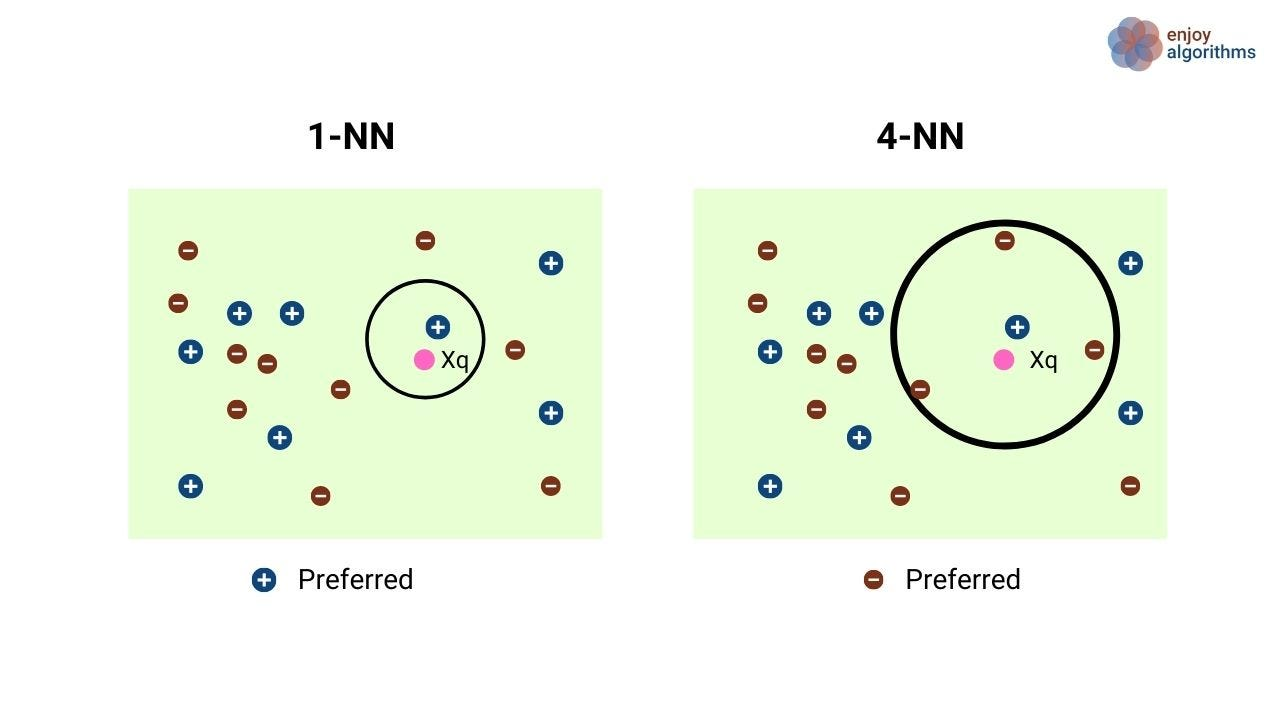Consider an example shown in the above image. Initially, the entire training dataset is considered and mapped in an R² space of positive and negative classes. The test case xq is then classified using 1-NN (1 neighbor) and 4-NN (4 neighbors) classifiers. The results for both are different, as we see that xq is classified as +ve for 1-NN and -ve for 4-NN.

### How the value of K affects the KNN algorithm?

The value of K in the KNN algorithm can be anything ranging from 1 to the total number of samples. A small value of K means that the model is overfitting and is vulnerable to outliers. This model will have high variance and low bias. On the other hand, a model with a high value of K will have low variance and high bias and will result in underfitting. When we slowly increase the value of K from 1 to the number of training samples, the model will start smoothing the boundary surfaces.

K = 1: A model with K=1 will have 0 training error and hard boundaries for determining the class of test query.

K = len(sample data): This model will be highly biased towards the majority class (with a higher number of samples) and less accurate.

Note: Keeping the K values as odd is advisable to reduce the chances of getting a tie.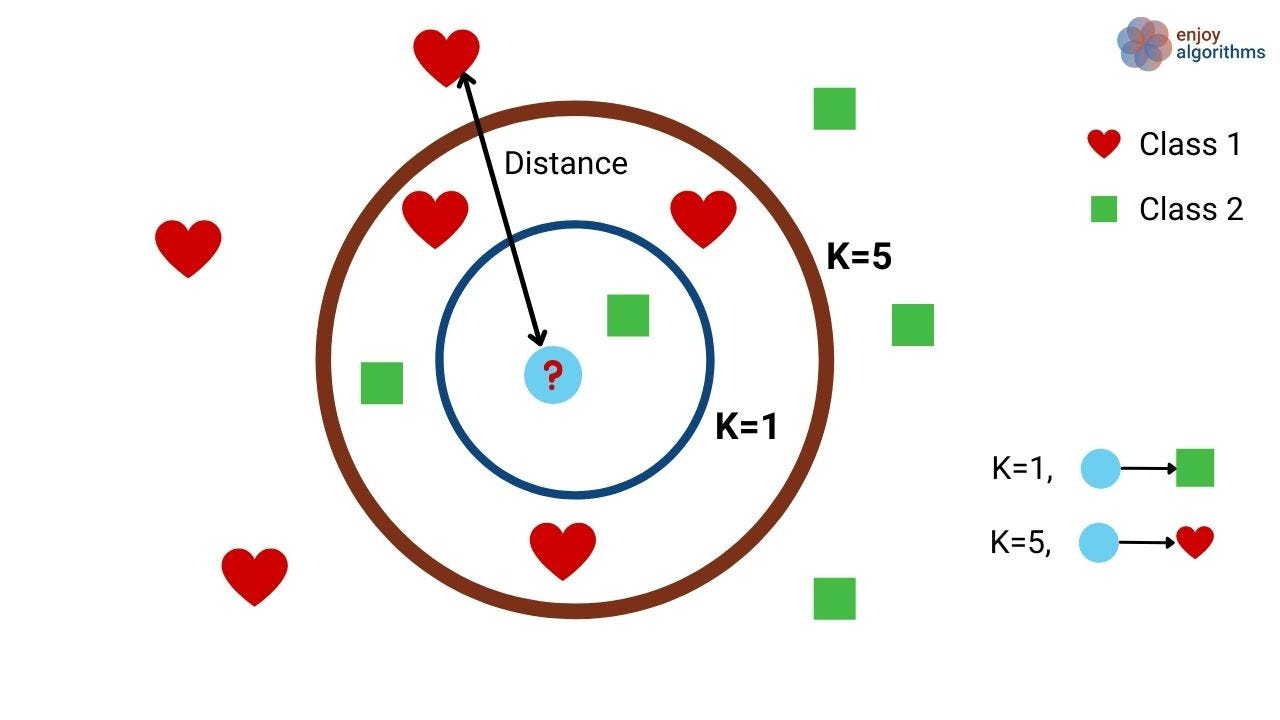### How does feature scaling affect KNN?

KNN depends highly on the distance between data samples; hence scaling plays a vital role here. Suppose we train the KNN algorithm on unscaled data. There can be a case where different attributes lie in various scales, making our model biased towards the features with lesser magnitude values. To avoid that, it is always advisable to standardize the attributes before applying the KNN algorithm. Please look at this blog to visualize how distance calculation can be affected by scaling for a better understanding.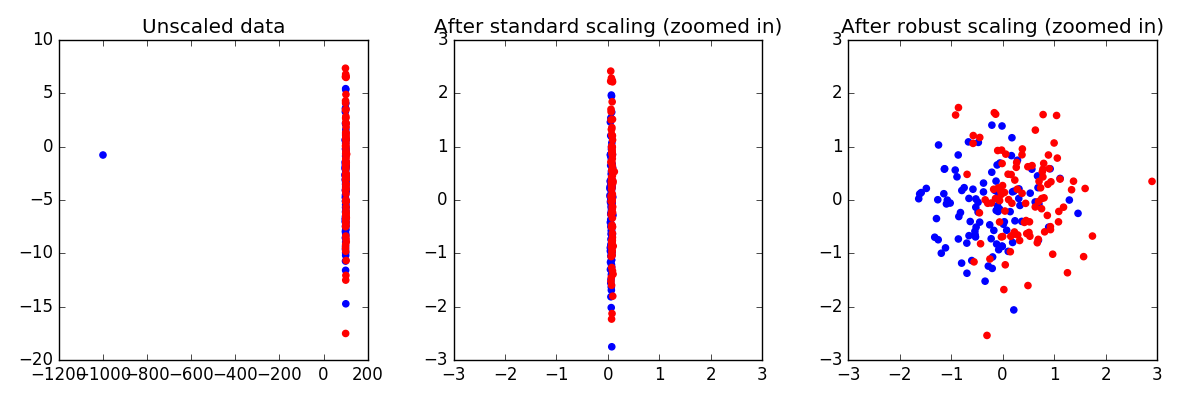### What are the Voronoi cell and Voronoi diagrams?

Other ML algorithms like linear regression, logistic regression, and SVMs try to fit a mapping function from input to output. This mapping function is also known as the Hypothesis function. But, KNN is different. It does not form any explicit Hypothesis function, but it does create a hypothesis space. For a dataset in R², the hypothesis space is a polyhedron formed using the training samples. Let's first understand what a Voronoi cell is.

#### What is Voronoi Cell?

Suppose the training set is "T" and the elements of that training set are "x". Then Voronoi Cell of xi is a polytope (a geometric shape with "flat" sides) consisting of all points closer to xi than any other points in T.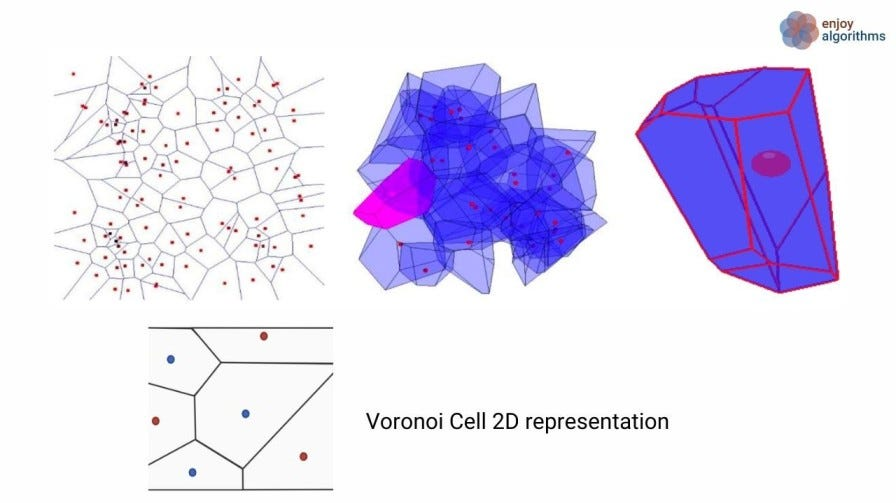If we observe in the above image, initially, every cell contains a single sample which means K = 0, and as we increase the value of K, two cells merge and form a new polytope including K samples. Voronoi Cells cover the entire training space of T, and when we combine all of these cells, it will create Voronoi Diagram.

### KNN for Regression problems

So far, we have discussed how we could use the KNN algorithm to solve the classification tasks, but this machine learning algorithm can also solve regression problems. We need to tweak the approach slightly. Instead of counting the K nearest neighbor class labels, what if we average the data over K neighbors. Yes! It will act as the regression model in such a scenario.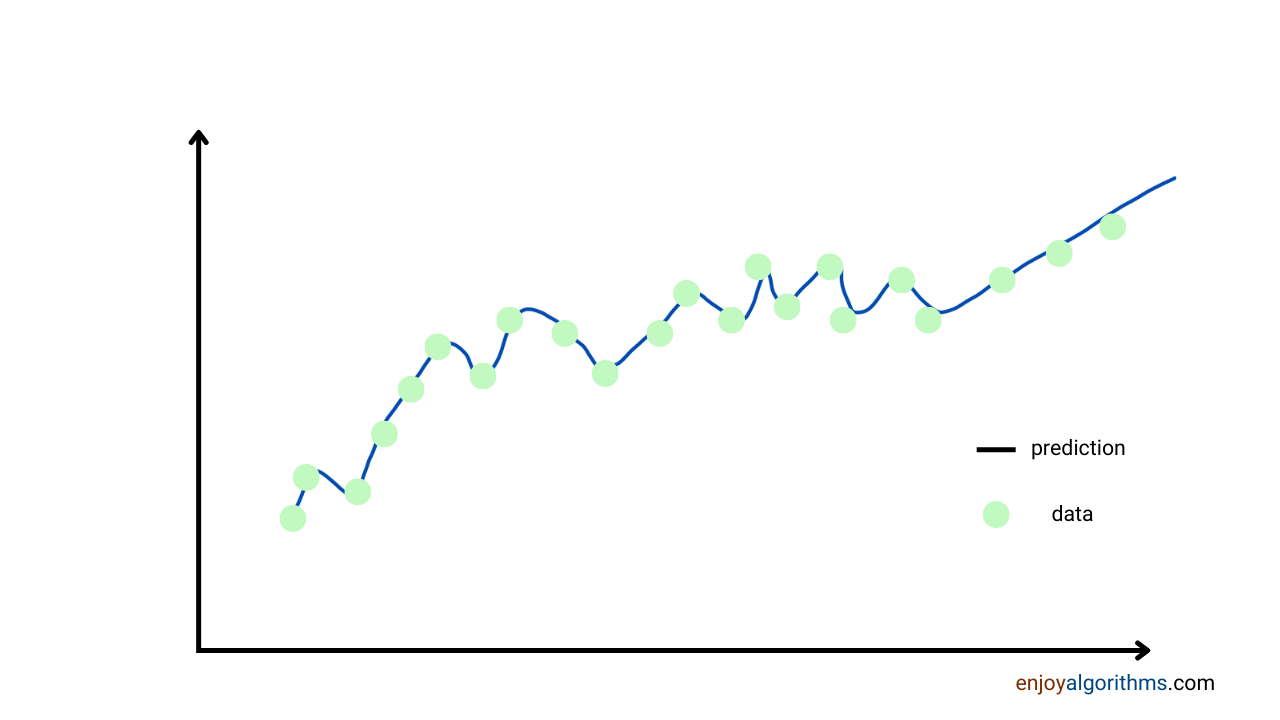For example, let's say we have a test data X for which we want to predict the continuous variable Y. Suppose we have finalized that our neighbors can only be 3 (i.e., K=3). Three neighbors from the training data are:

X1 → Y1, X2 → Y2, X3 → Y3. We should be clear that KNN is a supervised learning algorithm, and hence we will always have the corresponding labels for the input variables while training. At the time of prediction, we can average out the three labels to find the corresponding label of the test data. For example, Y = (Y1 + Y2 + Y3)/3. This averaging can be replaced with other techniques like median, mode, or any custom approach.

### Strengths of the KNN algorithm

KNN is a very famous algorithm because of its simplicity, so let's understand the key strengths.

1. Zero training time: A very little training time is required compared to the other machine learning algorithms.
2. Sample efficiency: There is no need for a very high training sample.
3. Explainable: At each step, the reason for the prediction can easily be depicted. Such explainability is rare.
4. Easy to add and remove the data: For other machine learning models, data addition requires retraining of the model. While in KNN, we can directly update the memory and perform the inference.
5. Less sensitive to class imbalance: Suppose we have two classes and one class has significantly higher instances in the dataset than the others. KNN, unlike other ML algorithms, is least affected by such class imbalances.

### Disadvantages of the KNN algorithm

No doubt, KNN is cool, but this algorithm has some limitations. It is not the first choice among Machine Learning experts, and the reasons are:

• Needs a lot of storage: KNN stores the whole training data in its memory and performs inference based on that. It makes the algorithm unemployable on edge platforms.
• Predictions are Slow: The time complexity of KNN is O(dN), where d is the dimension or number of features and N is the total number of samples. More the data more will be the prediction time.
• Irrelevant features can fool the nearest neighbors.

### KNN Implementation in Python using sklearn

Let's implement the KNN algorithm in python to solve a classification problem.

#### Step 1: Import the necessary dataset libraries.

The dataset used to implement KNN is the famous Iris dataset imported from the Scikit-learn datasets as load_iris. Other libraries are imported for training, preprocessing, and evaluation.

``````import matplotlib.pyplot as plt   # update the plot
from sklearn import datasets# read the data
import numpy as np #for arrays
from sklearn.model_selection import train_test_split # split the data
from sklearn.preprocessing import StandardScaler # scale the data
from sklearn.neighbors import KNeighborsClassifier # the algorithm

from sklearn.metrics import accuracy_score  #grade the results
import pandas as pd

X = iris.data[:]  # select the features to use
y = iris.target   # select the classes

iris_dataframe = pd.DataFrame (data= np.c_[iris['data'], iris['target']],

columns= iris['feature_names'] + ['target'])

plt.figure(2)
grr = pd.plotting.scatter_matrix(iris_dataframe,
c=iris["target"],
figsize=(15, 15),
marker='o',
S=60,
alpha=.8)
plt.show(2)
``````

#### Step 2: Understanding the data

This dataset has four variables: sepal length, sepal width, petal length, and petal width, describing iris plants of three types: Setosa, Versicolour, and Virginica. The dataset contains 150 observations, with each observation labeled as the actual type of the plant.

#### Step 3: Visualization

The dataset, which has four dimensions, is visualized pairwise to distinguish them. The pairwise scatter plot matrix of the iris data set helps visualize the relationship among multiple variables separately within subdivisions of the dataset. In the image below, violet color represents Setosa, green represents Versicolour, and yellow represents Virginica.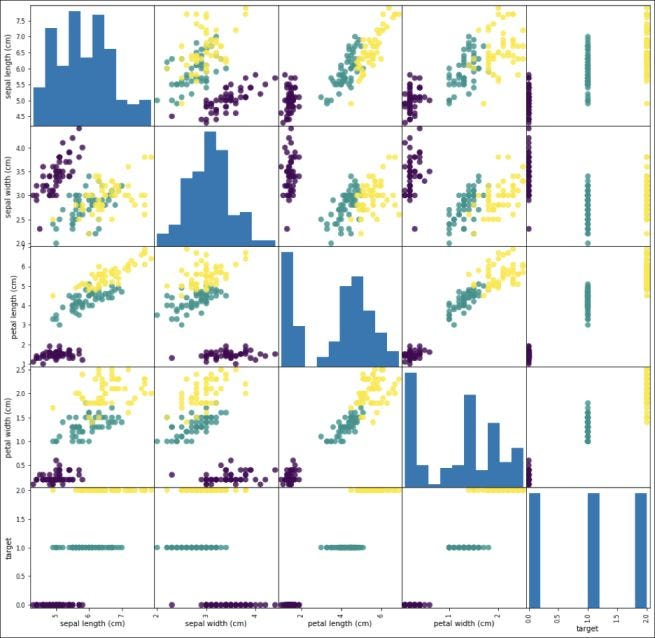#### Step 4: Data Preprocessing

The entire dataset is initially split into the training and testing part using the traintestsplit function of Scikit-learn. A standard scaler is used in the next step, StandardScalar( ), to standardize the data (column-wise). When fit to a dataset, the function will transform the dataset to mean μ = 0 and standard deviation σ = 1.

A dataset with having N samples and m features,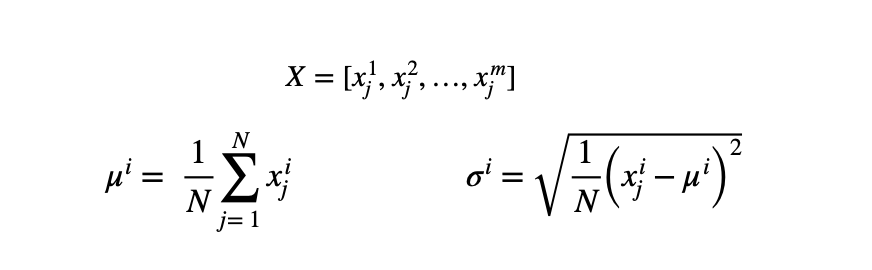Thus every data is then updated as,``````X_train, X_test, y_train, y_test = train_test_split(X,y, test_size=0.25,random_state=0)

SC = StandardScaler()# create the standard scaler
sc.fit(X_train) # fit to the training data
x_train_std = sc.transform(X_train) # transform the training data
X_test_std = sc.transform(X_test) # same transformation on test data
``````

#### Step 5: Model Fitting and Evaluation

We will fit the KNN model for different values of K ranging between 1 to the number of samples in the testing dataset. The metric "Minkowski" along with p = 2 represents the Euclidean distance in the R-space. The model will be fitted on different values of K and then is used to predict the output for a test sample size.

``````accuracyTest = {}; accuracy Train = {}

for k in range (len (y_test):

knn = KNeighborsClassifier(n_neighbors=k+1, p=2, metric='minkowski')
knn.fit(X_train_std,y_train)
y_pred = knn.predict(x_test_std)
y_train_pred = knn.predict(X_train_std)

if (k+1)%10==0:
print(10*'-')
print("For k = %s" %(k+1))
print('Number in test ', len(y_test))
print('Misclassified samples: %d' % (y_test != y_pred).sum())

accTrain = accuracy_score(y_train,y_train_pred)
acc = accuracy_score(y_test, y_pred)
accuracyTest[k+1] = acc
accuracyTrain[k+1] = accTrain

for accuracy in [accuracy Train, accuracy Test]:
lists = sorted(accuracy.items() # sorted by key, return a list of tuples
X, y = zip(*lists) # unpack a list of pairs into two tuples
plt.plot(x, y)
plt.show()
``````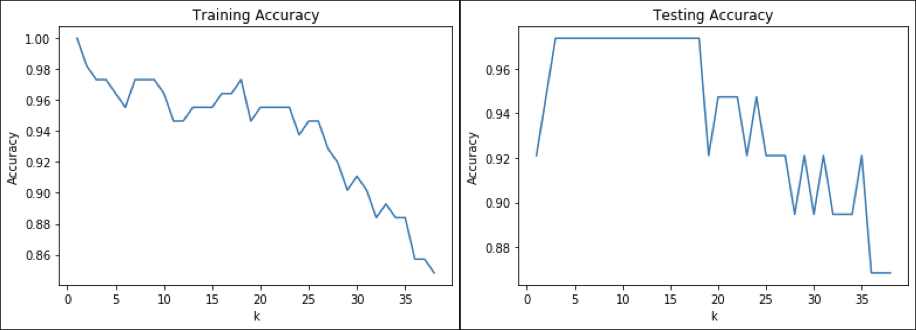If we give priority to the testing accuracy, the value of K > 18 decreases the testing accuracy sharply. So we can say that the optimal number of neighbors can be around 15 to 18.

### Decision Boundaries for KNN

The two datasets (training and testing) are combined to show the effect of varying K in the KNN algorithm. Only two features (petal length and petal width) are considered for visualization. The value of K taken is [1,25,50,75,100,112], where the training sample size is 112. The decision boundary at K = 112 returns the majority of the three classes, which is red.

``````X = iris.data[:, [2,3]] # select the features to use
y = iris.target         # select the classes

X_train, X_test, y_train, y_test = train_test_split(X,y, test_size=0.25,random_state=0)

SC = StandardScaler()# create the standard scaler
sc.fit(X_train) # fit to the training data
x_train_std = sc.transform(X_train) # transform the training data
X_test_std = sc.transform(X_test) # same transformation on test data

X_combined_std = np.vstack((X_train_std, X_test_std))
y_combined = np.hstack((y_train, y_test))

print('Number in combined ', len(y_combined))
# check results on combined data
y_combined_pred = knn.predict(X_combined_std)

print('Misclassified combined samples: %d' 1 % (y_combined != y combined_pred). sum )
print('Combined Accuracy: %.2f' % accuracy_score(y_combined, y_combined_pred))
# visualize the results

for k in [1,25,50, 100, len(X_train)]:

knn = KNeighborsClassifier (n_neighbors=k, p=2, metric='minkowski')

knn.fit(X_train_std, y_train)

plot_decision_regions(X=X_combined_std, y=y_combined, classifier=knn,
test_idx=range(105,150))

plt.xlabel('petal length [standardized]')
plt.ylabel('petal width [standardized]')
plt.title('k=%s'%k)
plt.legend(loc='upper left')
plt.show()
``````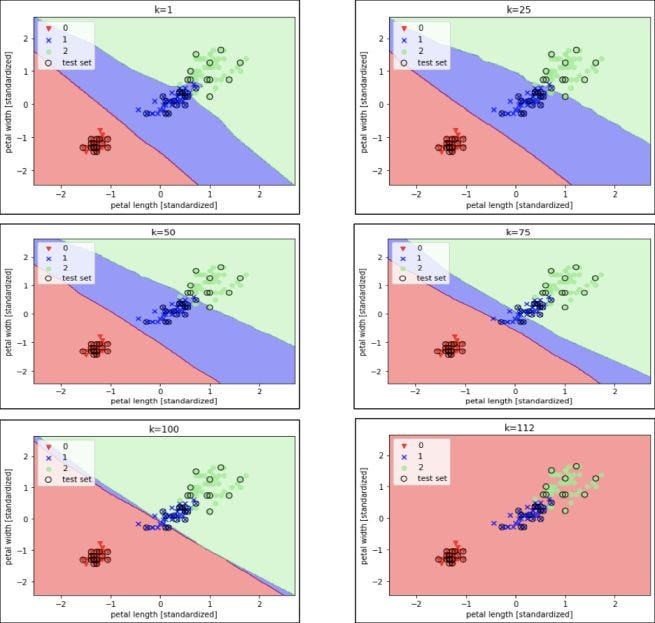### Industrial Applications of KNN

Although there are certain limitations, this algorithm is widely used in industries because of its simplicity. Some of these applications are:

• Email spam filtering: For detecting the trivial and fixed types of spam emails, KNN can perform well. The implementation steps of this algorithm can be found here.
• Wine Quality prediction: Wine quality prediction is a regression task and can be solved using the KNN algorithm. The implementation can be found here.
• Recommendation system: KNN is used to build the recommendation engines that recommend some products/movies/songs to the users based on their likings or disliking.

### Possible Interview Questions

As we stated, this algorithm brings a lot of explainability with itself. Interviewers can ask more profound questions on this topic. Some of them could be,

• How k-NN is different from other Machine Learning algorithms?
• Will changing the distance metric affect the classification accuracy?
• Is k-NN highly sensitive to data normalization?
• Why is it a non-parametric algorithm?
• What are the major cons of the k-NN algorithm?

### Conclusion

In this article, we have covered the concept of the first "Machine Learning" algorithm, i.e., KNearest Neighbour. We saw how we can define the instances as neighbors and how the value of K affects the predictions. We also discussed why feature scaling played a vital role and learned about the Voronoi Diagram. After that, we discussed the regression use-case of KNN. Finally, we implemented the KNN algorithm on the famous Iris dataset. We hope you enjoyed the article.

#### References

1. Scikit-learn: Machine Learning in Python, Pedregosa, et al., JMLR 12, pp. 2825–2830, 2011
2. Mitchell, T. M. (1997). Machine learning., McGraw Hill series in computer science New York: McGraw-Hill.
3. UCI Machine Learning Repository: Iris Data Set.
4. J. D. Hunter, “Matplotlib: A 2D Graphics Environment”, Computing in Science & Engineering, vol. 9, no. 3, pp. 90–95, 2007.

Enjoy Learning! Enjoy Algorithms!